>健康>正文

# 【ML】逻辑回归——详细概述

1 逻辑回归算法如何理解和应用？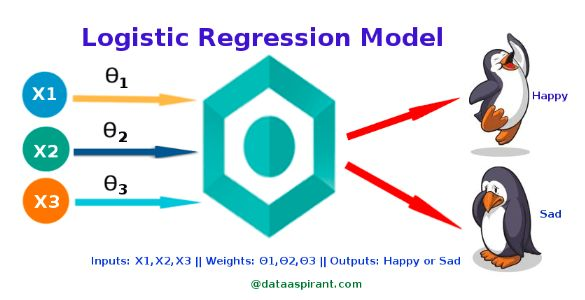• 预测电子邮件是垃圾邮件(1)还是(0)
• 肿瘤是否恶性(1)(0)

https://github.com/SSaishruthi/LogisticRegression_Vectorized_Implementation/blob/master/Logistic_Regression.ipynb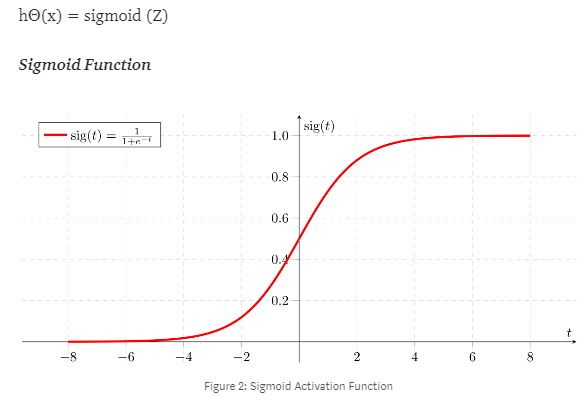X = [x0 x1] = [1 IP-Address]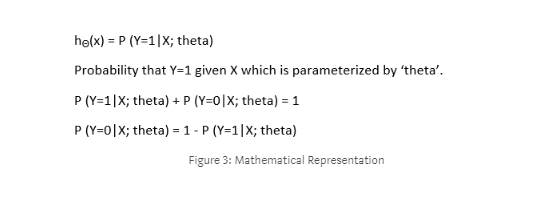1. 二元逻辑回归：分类反应只有两种可能结果。例子:垃圾邮件或非垃圾邮件
2. 多项逻辑回归：三个或更多的类别，没有排序。例子:预测哪种食物更受欢迎(素食，非素食，纯素食)
3. 顺序逻辑回归：三个或更多类别的排序。例子:电影分级从1到5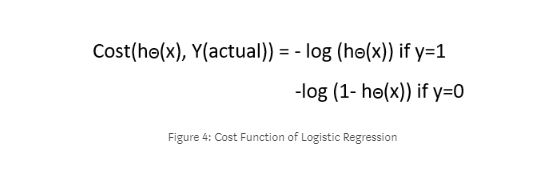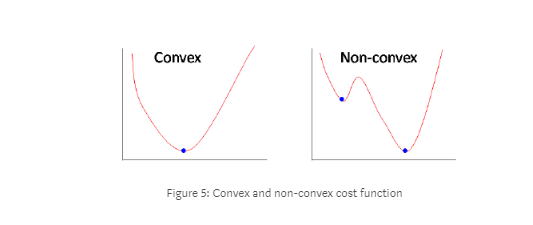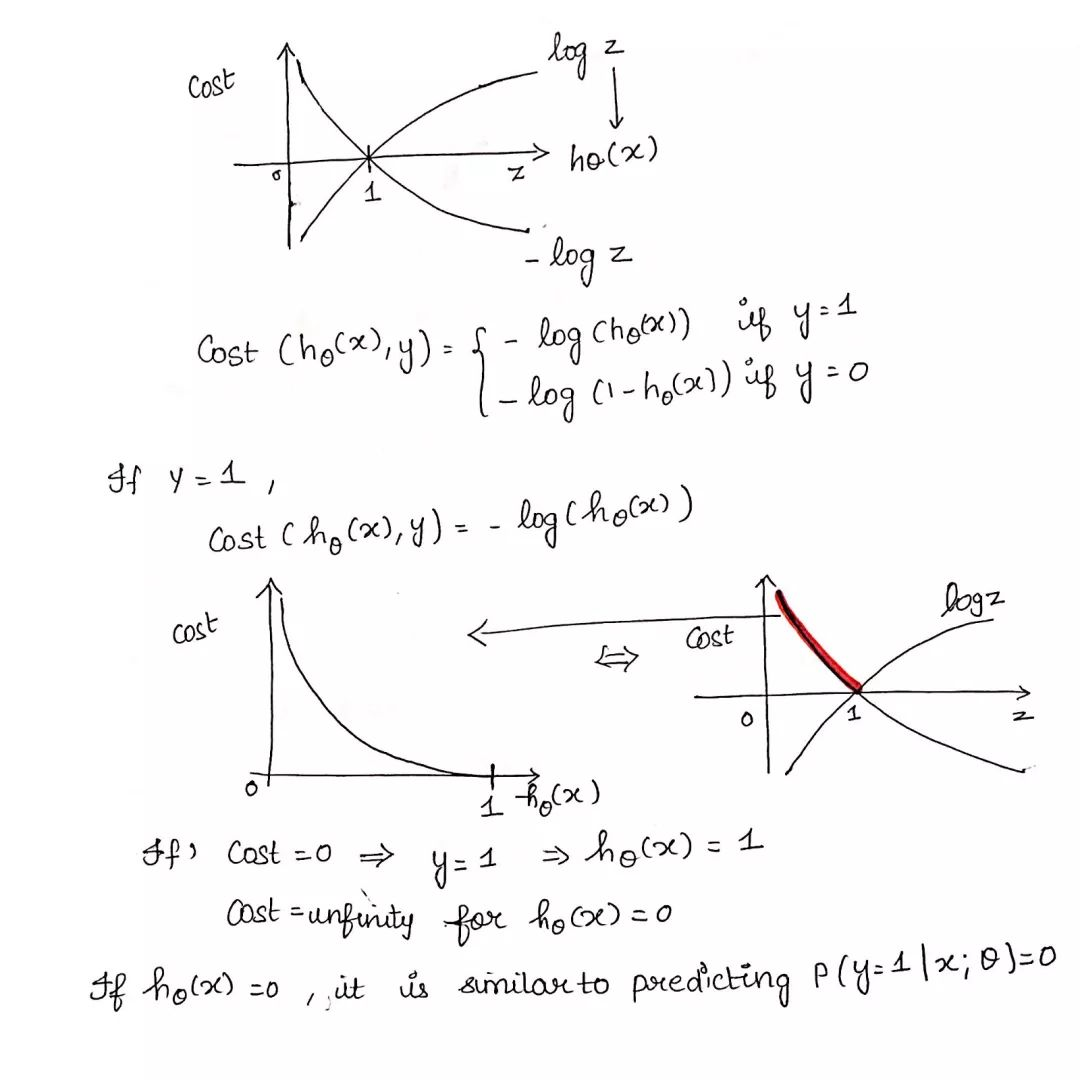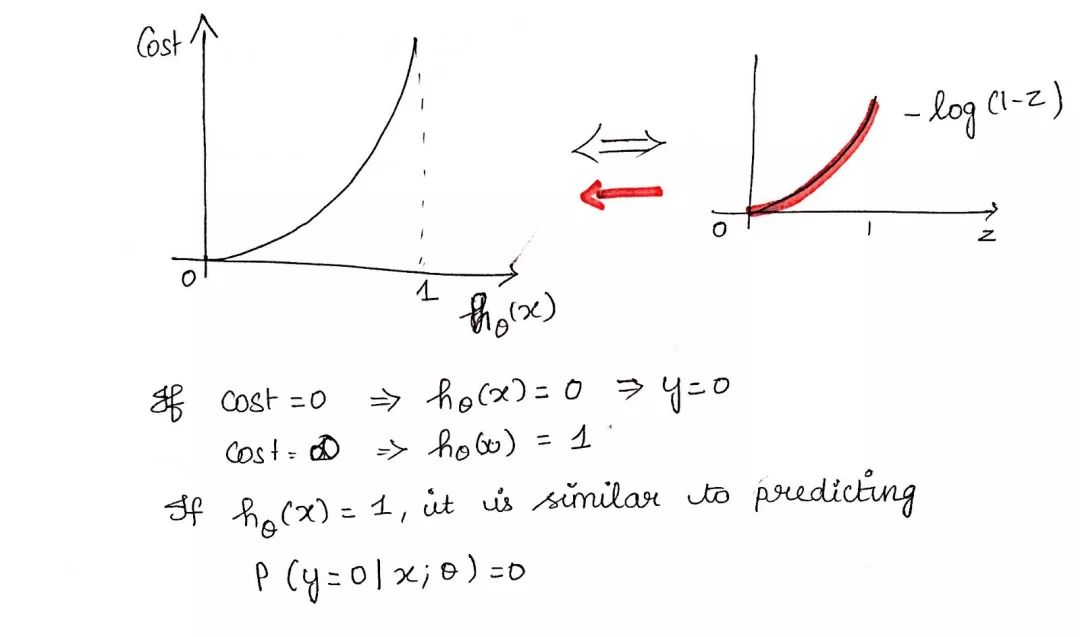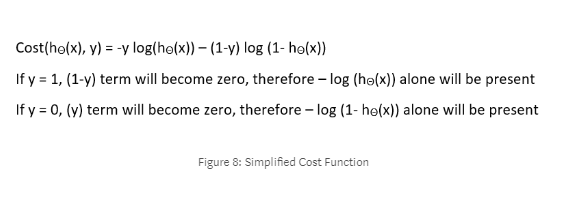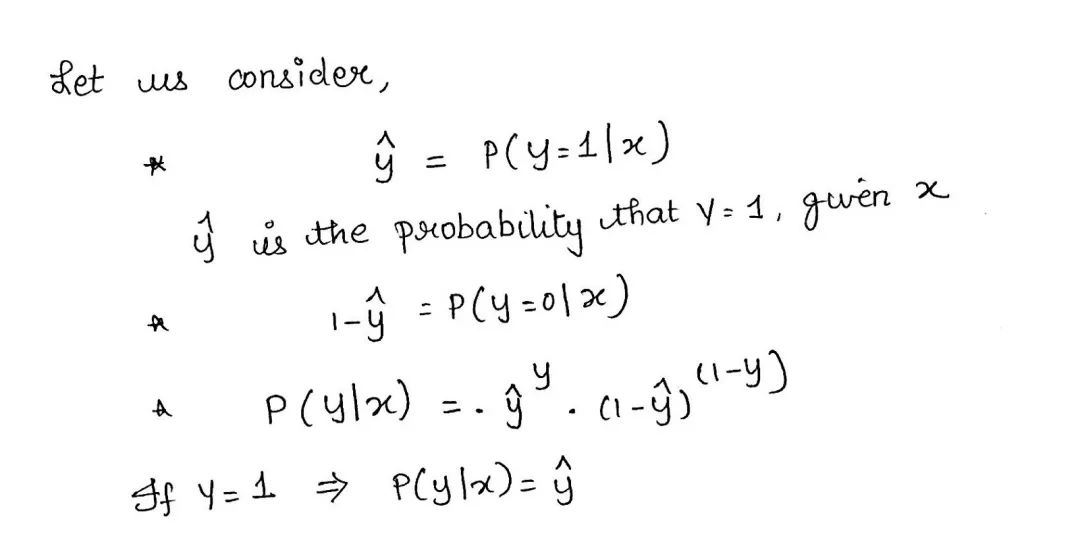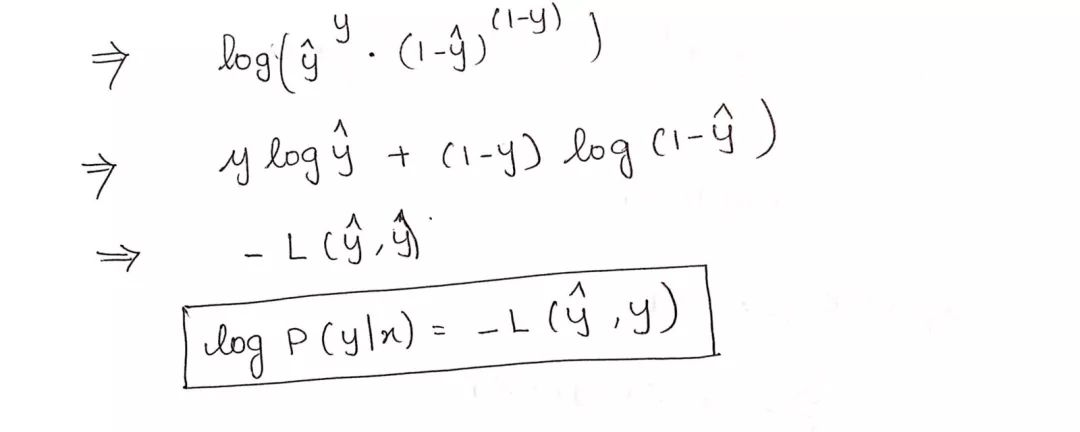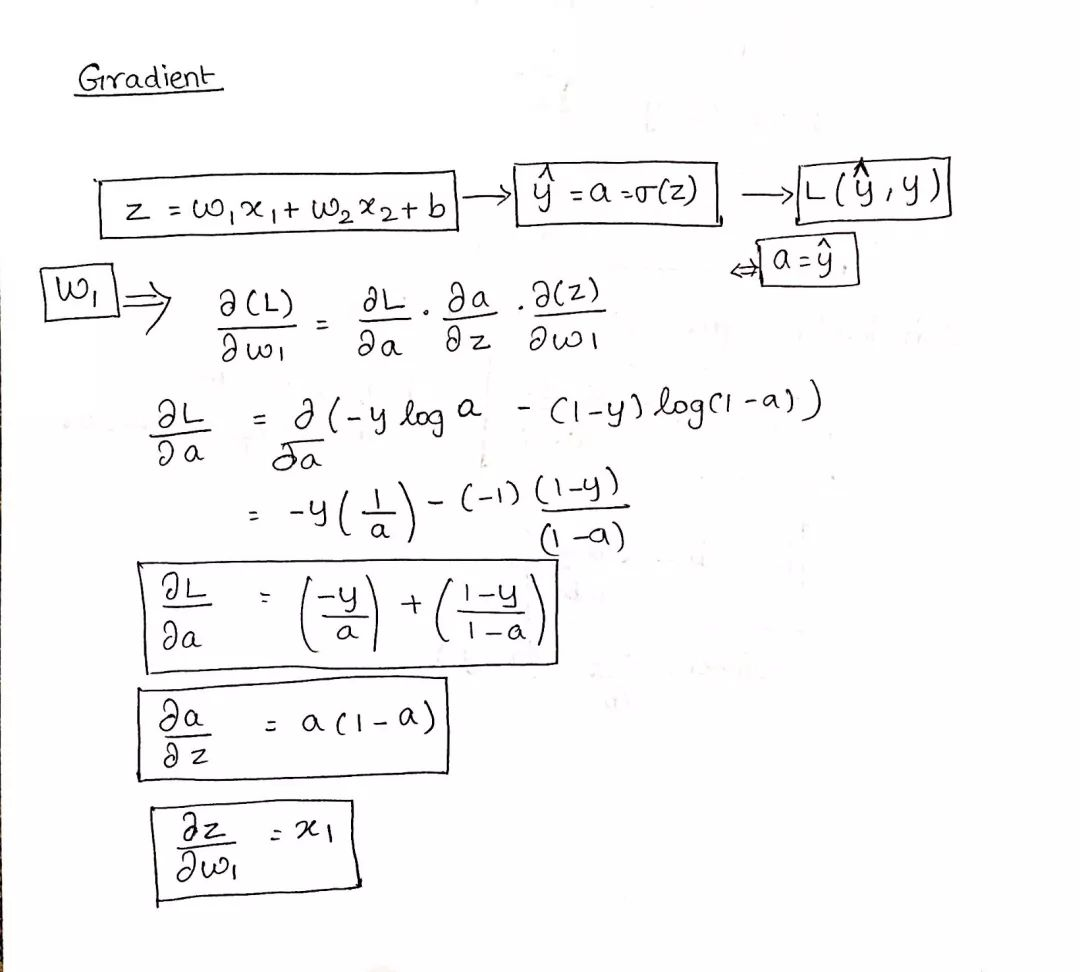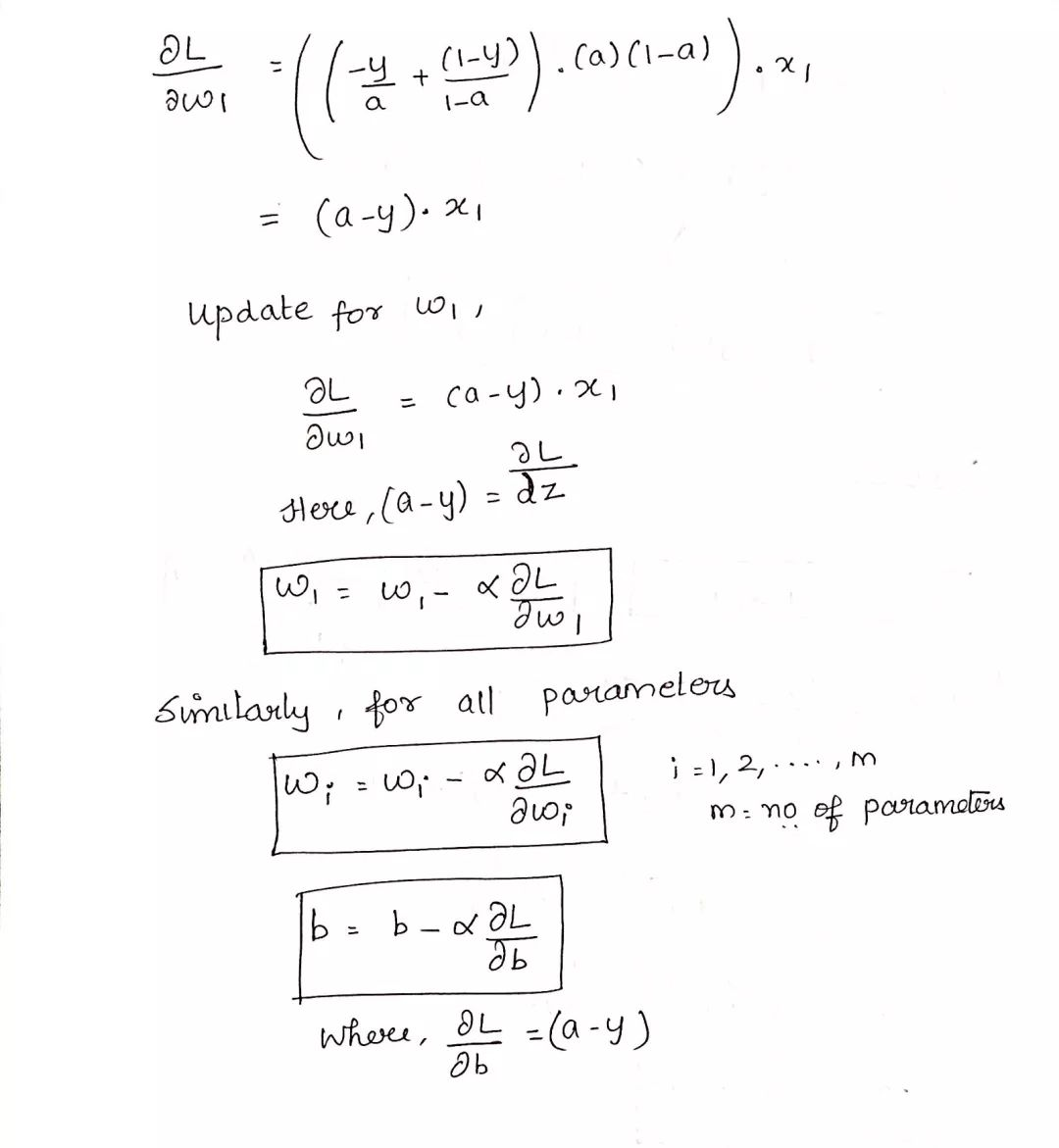Python实现1defweightInitialization(n_features):

2w = np.zeros(( 1,n_features))

3b = 0

4returnw,b

5defsigmoid_activation(result):

6final_result = 1/( 1+np.exp(-result))

7returnfinal_result

8

9defmodel_optimize(w, b, X, Y):

10m = X.shape[ 0]

11

12#Prediction

13final_result = sigmoid_activation(np.dot(w,X.T)+b)

14Y_T = Y.T

15cost = ( -1/m)*(np.sum((Y_T*np.log(final_result)) + (( 1-Y_T)*(np.log( 1-final_result)))))

16#

17

19dw = ( 1/m)*(np.dot(X.T, (final_result-Y.T).T))

20db = ( 1/m)*(np.sum(final_result-Y.T))

21

22grads = { "dw": dw, "db": db}

23

25defmodel_predict(w, b, X, Y, learning_rate, no_iterations):

26costs = []

27fori inrange(no_iterations):

28#

29grads, cost = model_optimize(w,b,X,Y)

30#

31dw = grads[ "dw"]

32db = grads[ "db"]

33#weight update

34w = w - (learning_rate * (dw.T))

35b = b - (learning_rate * db)

36#

37

38if(i % 100== 0):

39costs.append(cost)

40#print("Cost after %i iteration is %f" %(i, cost))

41

42#final parameters

43coeff = { "w": w, "b": b}

44gradient = { "dw": dw, "db": db}

45

47defpredict(final_pred, m):

48y_pred = np.zeros(( 1,m))

49fori inrange(final_pred.shape[ 1]):

50iffinal_pred[ 0][i] > 0.5:

51y_pred[ 0][i] = 1

52returny_pred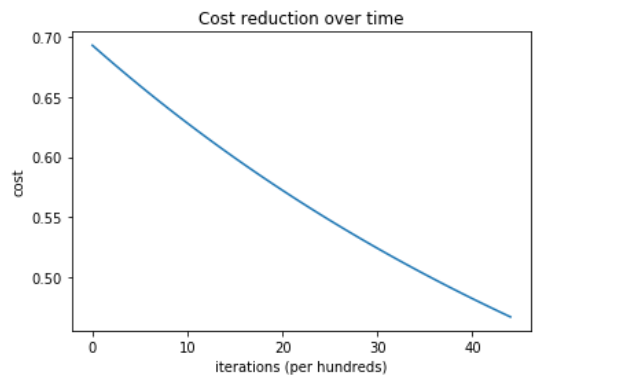https://github.com/SSaishruthi/LogisticRegression_Vectorized_Implementation/blob/master/Logistic_Regression.ipynb

https://towardsdatascience.com/logistic-regression-detailed-overview-46c4da4303bc